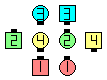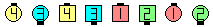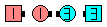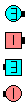Definitions of Square Dance Calls and Concepts
Strut Right | Left (And Right | Left) [C3B]Index -->  Plus  |  A1  |  A2  |  C1  |  C2  |  C3A  |  C3B  |  C4  |  NOL  |
Definitions (Text Only) -->  Plus  |  A1  |  A2  |  C1  |  C2  |  C3A  |  C3B  |  C4  |  NOL  |
 Find call:

Strut Right | Left (And Right | Left) -- [C3B]
(Lee Kopman 1977)

 \$B8@8l(BFrom a Double Pass Thru or a R-H 1/4 Tag. EN: 10
Double Pass Thru \$B\$^\$?\$O(B R-H 1/4 Tag \$B\$+\$i(B. JP: 10

Centers Pass Thru, Right | Left Turn To A Line and Spread to become #1 (Very End) and #3 in a Tidal Line as others Step Ahead and Veer Right | Left to become #2 and #4 in a Tidal Line. EN: 20
Centers \$B\$O(B Pass Thru \$B\$r\$7\$F(B, Right | Left Turn To A Line and Spread \$B\$r\$7(B Tidal Line \$B\$N(B #1 (Very End) \$B\$H(B #3 \$B\$H\$J\$j(B, \$BB>\$N?M\$O(B Step Ahead and Veer Right | Left \$B\$r\$7(B Tidal Line \$B\$N(B #2 \$B\$H(B #4 \$B\$K\$J\$j\$^\$9(B. JP: 20Strut Right\$B\$NA0(B \$B8e(B

\$BCm

• If two directions are given, the original Centers take the first direction and the original Outsides take the second direction. If only one direction is given, everybody takes that direction. EN: 30
\$B\$b\$7(B 2 \$B\$D\$N(B direction \$BM?\$(\$i\$l\$?\$H\$-\$O(B, \$B%*%j%8%J%k\$N(B Centers \$B\$,(B 1 \$B\$DL\\$N(B direction \$B\$r(B, \$B%*%j%8%J%k\$N(B Outsides \$B\$O(B 2 \$B\$DL\\$N(B direction \$B\$r;H\$\$\$^\$9(B. \$B\$b\$7(B 1 \$B\$D\$@\$1\$N(B direction \$B\$,M?\$(\$i\$l\$?\$H\$-\$O(B, \$BA40w\$G\$=\$N(B direction \$B\$r;H\$\$\$^\$9(B. JP: 30
• The Centers always pass Right shoulders even if the call is Strut Left. The caller should say Left Strut Right|Left if a Left shoulder pass is desired. EN: 40
Centers \$B\$O(B, \$B%3!<%k\$,(B Strut Left \$B\$G\$"\$C\$F\$b(B, \$B>o\$K(B\$B1&(B\$B8*\$GDL\$j\$^\$9(B. \$B\$b\$7(B, \$B:8(B\$B8*\$GDL\$j\$?\$\$\$J\$i(B, \$B%3!<%i!<\$O(B Left Strut Right|Left \$B\$H8@\$o\$J\$1\$l\$P\$J\$j\$^\$;\$s(B. JP: 40
• If your given direction is Right, then you must end as a Beau (i.e., you want to end as if you are in a Right-hand Tidal Wave); Similarly, if your given direction is Left, you must end as a BelleEN: 50
\$B\$b\$7(B, \$B\$"\$J\$?\$XM?\$(\$i\$l\$?(B direction \$B\$,(B Right \$B\$N\$H\$-\$O(B, Beau \$B\$H\$J\$k(B (\$B\$9\$J\$o\$A(B, \$B<+J,\$N0LCV\$O(B Right-hand Tidal Wave \$B\$K\$\$\$k\$h\$&\$K(B) \$B\$O\$:\$G\$9(B. \$BF1MM\$K(B, \$BM?\$(\$i\$l\$?(B direction \$B\$,(B Left \$B\$N\$H\$-\$O(B, Belle \$B\$G\$H\$J\$k\$O\$:\$G\$9(B. JP: 50
• Hint: The original Outsides should not move until the Centers have almost completed their part of the call, so that sufficient space is available for the Outsides to execute their part. EN: 60
\$B%R%s%H(B: \$B%*%j%8%J%k\$N(B Outsides \$B\$O(B, Centers \$B\$,%3!<%k\$r=*\$(\$kD>A0\$^\$G(B, \$BF0\$-;O\$a\$k\$Y\$-\$G\$O\$"\$j\$^\$;\$s(B. \$B\$3\$N\$3\$H\$G(B, Outsides \$B\$,F0\$/\$?\$a\$N==J,\$J>l=j\$,F@\$i\$l\$^\$9(B. JP: 60

Single Strut Right | Left (And Right | Left) [C3B] (Lee Kopman 1977):
From a Single Double Pass Thru, a Single R-H 1/4 Tag, or other applicable formations. Centers Pass Thru, Single Right|Left Turn To A Line and Spread as the others Step Ahead and Single Veer Right|Left. Usually ends in a Wave or Line. EN: 825
Single Double Pass Thru, Single R-H 1/4 Tag, \$B\$^\$?\$OB>\$NE,@Z\$J(B formation \$B\$+\$i(B. Centers \$B\$O(B Pass Thru \$B\$r\$7\$F(B, Single Right|Left Turn To A Line and Spread \$B\$r\$7(B, \$BB>\$N?M\$O(B Step Ahead and Single Veer Right|Left \$B\$r\$7\$^\$9(B. \$BIaDL(B Wave \$B\$^\$?\$O(B Line \$B\$G=*\$o\$j\$^\$9(B. JP: 825Single Strut Right And Left\$B\$NA0(B \$B8e(BChoreography for Strut Right | Left (And Right | Left)Comments? Questions? Suggestions?